scatter

•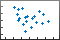Description

Vector and Matrix Data

example

scatter(x,y) creates a scatter plot with circular markers at the locations specified by the vectors x and y.

• To plot one set of coordinates, specify x and y as vectors of equal length.

• To plot multiple sets of coordinates on the same set of axes, specify at least one of x or y as a matrix.

example

scatter(x,y,sz) specifies the circle sizes. To use the same size for all the circles, specify sz as a scalar. To plot each circle with a different size, specify sz as a vector or a matrix.

example

scatter(x,y,sz,c) specifies the circle colors. You can specify one color for all the circles, or you can vary the color. For example, you can plot all red circles by specifying c as 'red'.

example

scatter(___,'filled') fills in the circles. Use the 'filled' option with any of the input argument combinations in the previous syntaxes.

example

scatter(___,mkr) specifies the marker type.

Table Data

example

scatter(tbl,xvar,yvar) plots the variables xvar and yvar from the table tbl. To plot one data set, specify one variable for xvar and one variable for yvar. To plot multiple data sets, specify multiple variables for xvar, yvar, or both. If both arguments specify multiple variables, they must specify the same number of variables. (Since R2021b)

example

scatter(tbl,xvar,yvar,'filled') plots the specified variables from the table with filled circles. (Since R2021b)

example

scatter(ax,___) plots into the axes specified by ax instead of into the current axes. The option ax can precede any of the input argument combinations in the previous syntaxes.

example

scatter(___,Name,Value) modifies the scatter plot using one or more name-value arguments to set properties. For example:

• scatter(x,y,'LineWidth',2) creates a scatter plot with 2-point marker outlines.

• scatter(tbl,'MyX','MyY','ColorVariable','MyColors') creates a scatter plot from data in a table, and customizes the marker colors using data from the table.

For a full list of properties, see Scatter Properties.

example

s = scatter(___) returns the Scatter object or an array of Scatter objects. Use s to set properties after creating the plot. For a full list of properties, see Scatter Properties.

Examples

collapse all

Create x as 200 equally spaced values between 0 and $3\pi$. Create y as cosine values with random noise. Then, create a scatter plot.

x = linspace(0,3*pi,200);
y = cos(x) + rand(1,200);
scatter(x,y)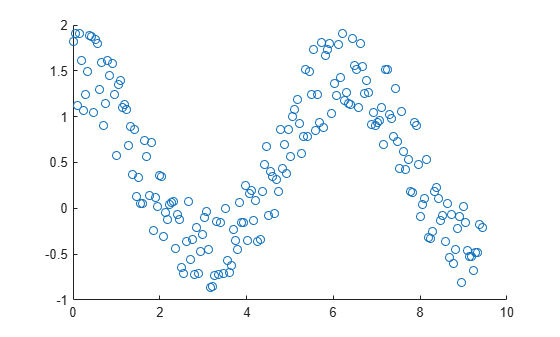Create a scatter plot using circles with different sizes. Specify the size in points squared

x = linspace(0,3*pi,200);
y = cos(x) + rand(1,200);
sz = linspace(1,100,200);
scatter(x,y,sz)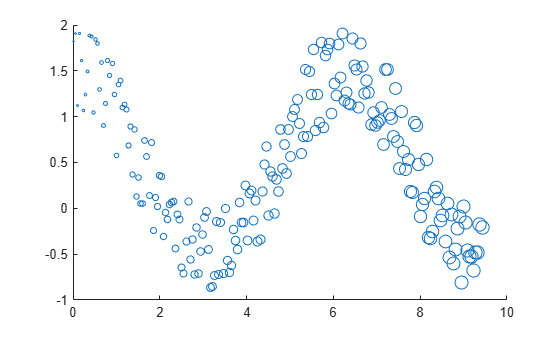Corresponding elements in x, y, and sz determine the location and size of each circle. To plot all circles with the equal area, specify sz as a numeric scalar.

Create a scatter plot and vary the circle color.

x = linspace(0,3*pi,200);
y = cos(x) + rand(1,200);
c = linspace(1,10,length(x));
scatter(x,y,[],c)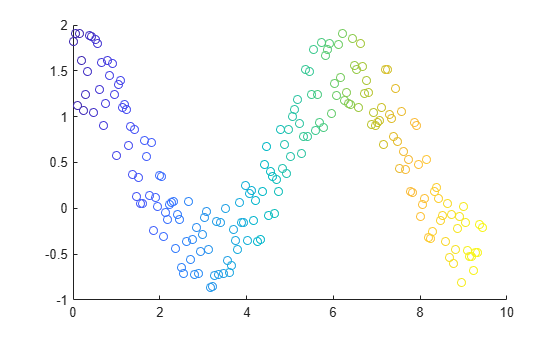Corresponding elements in x, y, and c determine the location and color of each circle. The scatter function maps the elements in c to colors in the current colormap.

Create a scatter plot and fill in the markers. scatter fills each marker using the color of the marker edge.

x = linspace(0,3*pi,200);
y = cos(x) + rand(1,200);
sz = 25;
c = linspace(1,10,length(x));
scatter(x,y,sz,c,'filled')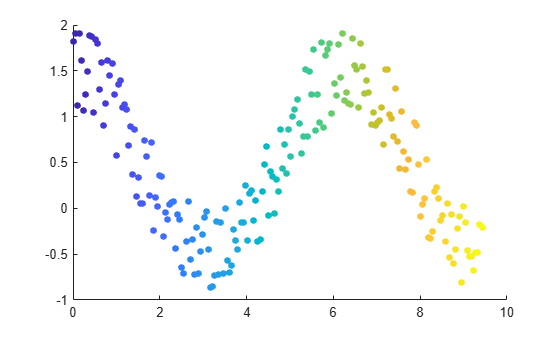Create vectors x and y as sine and cosine values with random noise. Then, create a scatter plot and use diamond markers with an area of 140 points squared.

theta = linspace(0,2*pi,150);
x = sin(theta) + 0.75*rand(1,150);
y = cos(theta) + 0.75*rand(1,150);
sz = 140;
scatter(x,y,sz,'d')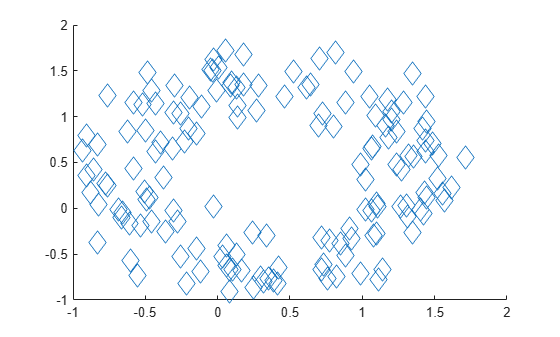Create vectors x and y as sine and cosine values with random noise. Create a scatter plot and set the marker edge color, marker face color, and line width.

theta = linspace(0,2*pi,300);
x = sin(theta) + 0.75*rand(1,300);
y = cos(theta) + 0.75*rand(1,300);
sz = 40;
scatter(x,y,sz,'MarkerEdgeColor',[0 .5 .5],...
'MarkerFaceColor',[0 .7 .7],...
'LineWidth',1.5)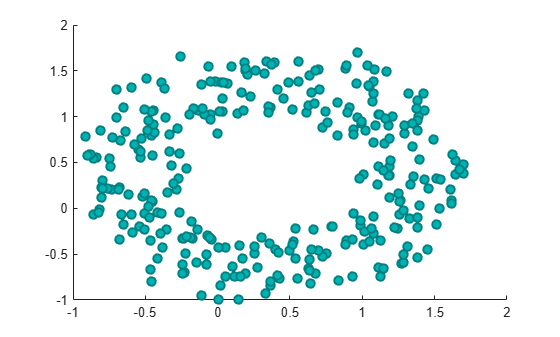You can vary the transparency of scattered points by setting the AlphaData property to a vector of different opacity values. To ensure the scatter plot uses the AlphaData values, set the MarkerFaceAlpha property to 'flat'.

Create a set of normally distributed random numbers. Then create a scatter plot of the data with filled markers.

x = randn(1000,1);
y = randn(1000,1);
s = scatter(x,y,'filled');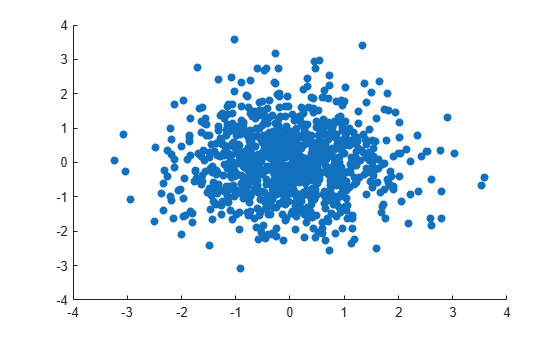Set the opacity of each point according to its distance from zero.

distfromzero = sqrt(x.^2 + y.^2);
s.MarkerFaceAlpha = 'flat';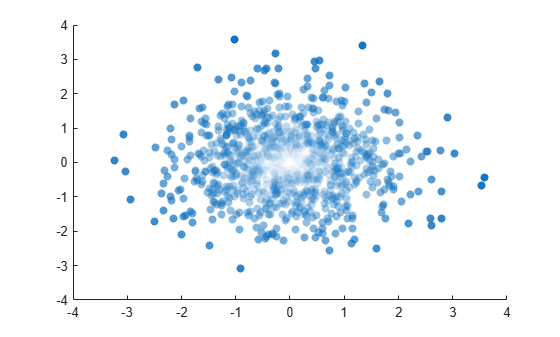Since R2021b

A convenient way to plot data from a table is to pass the table to the scatter function and specify the variables you want to plot. For example, read patients.xls as a table tbl. Plot the relationship between the Systolic and Diastolic variables by passing tbl as the first argument to the scatter function followed by the variable names. Notice that the axis labels match the variable names.

scatter(tbl,'Systolic','Diastolic');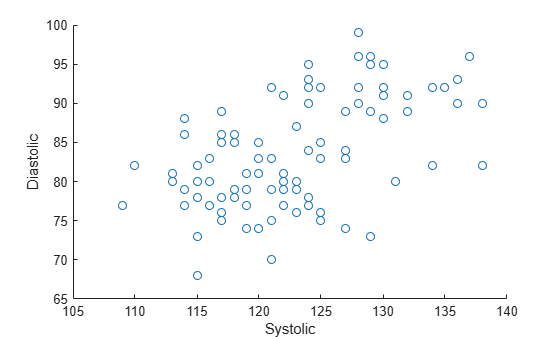You can also plot multiple variables at the same time. For example, plot both blood pressure variables versus the Weight variable by specifying the yvar argument as the cell array {'Systolic','Diastolic'}. Add a legend, and notice that the legend labels match the variable names.

scatter(tbl,'Weight',{'Systolic','Diastolic'});
legend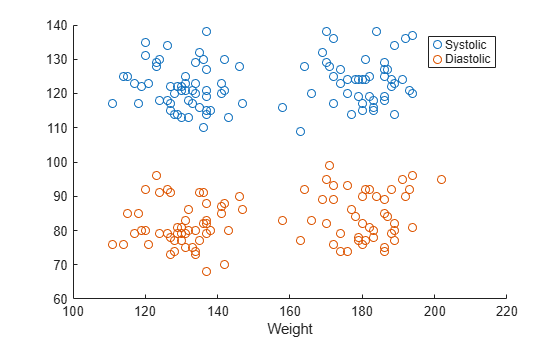Since R2021b

One way to plot data from a table and customize the colors and marker sizes is to set the ColorVariable and SizeData properties. You can set these properties as name-value arguments when you call the scatter function, or you can set them on the Scatter object later.

For example, read patients.xls as a table tbl. Plot the Height variable versus the Weight variable with filled markers. Vary the marker colors by specifying the ColorVariable name-value argument. Return the Scatter object as s, so you can set other properties later.

s = scatter(tbl,'Weight','Height','filled','ColorVariable','Diastolic');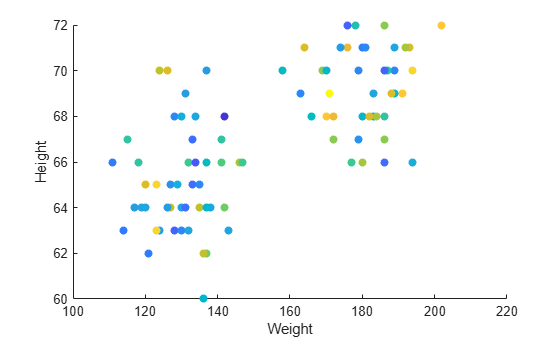Change the marker sizes to 100 points by setting the SizeData property. Then add a colorbar.

s.SizeData = 100;
colorbar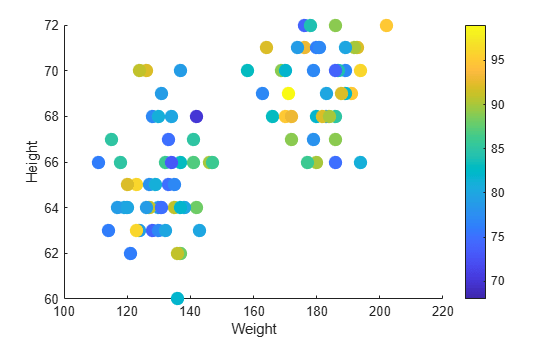Starting in R2019b, you can display a tiling of plots using the tiledlayout and nexttile functions. Call the tiledlayout function to create a 2-by-1 tiled chart layout. Call the nexttile function to create the axes objects ax1 and ax2. Plot scattered data into each axes. In the bottom scatter plot, specify diamond filled diamond markers.

x = linspace(0,3*pi,200);
y = cos(x) + rand(1,200);
tiledlayout(2,1)

% Top plot
ax1 = nexttile;
scatter(ax1,x,y)

% Bottom plot
ax2 = nexttile;
scatter(ax2,x,y,'filled','d')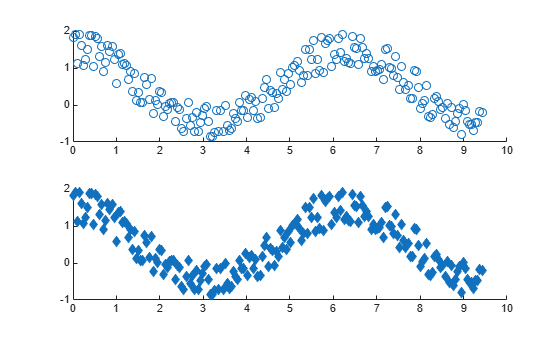Create a scatter plot and return the scatter series object, s.

theta = linspace(0,1,500);
x = exp(theta).*sin(100*theta);
y = exp(theta).*cos(100*theta);
s = scatter(x,y);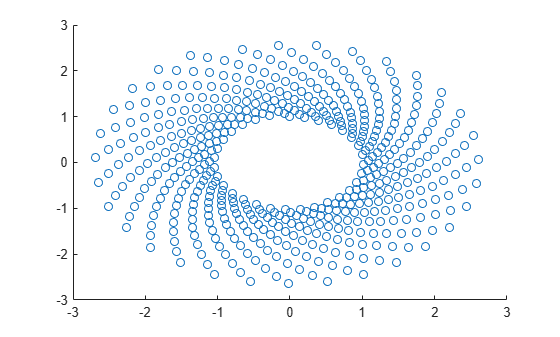Use s to query and set properties of the scatter series after it has been created. Set the line width to 0.6 point. Set the marker edge color to blue. Set the marker face color using an RGB triplet color.

s.LineWidth = 0.6;
s.MarkerEdgeColor = 'b';
s.MarkerFaceColor = [0 0.5 0.5];Input Arguments

collapse all

x-coordinates, specified as a scalar, vector, or matrix. The size and shape of x depends on the shape of your data. This table describes the most common situations.

Type of PlotHow to Specify Coordinates
Single point

Specify x and y as scalars. For example:

scatter(1,2)

One set of points

Specify x and y as any combination of row or column vectors of the same length. For example:

scatter([1 2 3],[4; 5; 6])

Multiple sets of points that are different colors

If all the sets share the same x- or y-coordinates, specify the shared coordinates as a vector and the other coordinates as a matrix. The length of the vector must match one of the dimensions of the matrix. For example:

scatter([1 2 3],[4 5 6; 7 8 9])
If the matrix is square, scatter plots a separate set of points for each column in the matrix.

Alternatively, specify x and y as matrices of equal size. In this case, scatter plots each column of y against the corresponding column of x. For example:

scatter([1 3 5; 2 4 6],[10 25 45; 20 40 60])

Data Types: single | double | int8 | int16 | int32 | int64 | uint8 | uint16 | uint32 | uint64 | categorical | datetime | duration

y-coordinates, specified as a scalar, vector, or matrix. The size and shape of y depends on the shape of your data. This table describes the most common situations.

Type of PlotHow to Specify Coordinates
Single point

Specify x and y as scalars. For example:

scatter(1,2)

One set of points

Specify x and y as any combination of row or column vectors of the same length. For example:

scatter([1 2 3],[4; 5; 6])

Multiple sets of points that are different colors

If all the sets share the same x- or y-coordinates, specify the shared coordinates as a vector and the other coordinates as a matrix. The length of the vector must match one of the dimensions of the matrix. For example:

scatter([1 2 3],[4 5 6; 7 8 9])
If the matrix is square, scatter plots a separate set of points for each column in the matrix.

Alternatively, specify x and y as matrices of equal size. In this case, scatter plots each column of y against the corresponding column of x. For example:

scatter([1 3 5; 2 4 6],[10 25 45; 20 40 60])

Data Types: single | double | int8 | int16 | int32 | int64 | uint8 | uint16 | uint32 | uint64 | categorical | datetime | duration

Marker size, specified as a numeric scalar, vector, matrix, or empty array ([]). The size controls the area of each marker in points squared. An empty array specifies the default size of 36 points. The way you specify the size depends on how you specify x and y, and how you want the plot to look. This table describes the most common situations.

Desired Marker Sizesx and y szExample

Same size for all points

Any valid combination of vectors or matrices described for x and y.

Scalar

Specify x as a vector, y as a matrix, and sz as a scalar.

x = [1 2 3 4];
y = [1 6; 3 8; 2 7; 4 9];
scatter(x,y,100)

Different size for each point

Vectors of the same length

• A vector with the same length as x and y.

• A matrix with at least one dimension that matches the lengths of x and y. Specifying a matrix is useful for displaying multiple markers with different sizes at each (x,y) location.

Specify x, y, and sz as vectors.

x = [1 2 3 4];
y = [1 3 2 4];
sz = [80 150 700 50];
scatter(x,y,sz)

Specify x and y as vectors and sz as a matrix.

x = [1 2 3 4];
y = [1 3 2 4];
sz = [80 30; 150 900; 50 2000; 200 350];
scatter(x,y,sz)

Different size for each point

At least one of x or y is a matrix for plotting multiple data sets

• A vector with the same number of elements as there are points in each data set.

• A matrix that has the same size as the x or y matrix.

Specify x as a vector, y as a matrix, and sz as vector.

x = [1 2 3 4];
y = [1 6; 3 8; 2 7; 4 9];
sz = [80 150 50 700];
scatter(x,y,sz)

Specify x as a vector, y as a matrix, and sz as a matrix the same size as y.

x = [1 2 3 4];
y = [1 6; 3 8; 2 7; 4 9];
sz = [80 30; 150 900; 50 2000; 200 350];
scatter(x,y,sz)

Marker color, specified as a color name, RGB triplet, matrix of RGB triplets, or a vector of colormap indices.

• Color name — A color name such as 'red', or a short name such as 'r'.

• RGB triplet — A three-element row vector whose elements specify the intensities of the red, green, and blue components of the color. The intensities must be in the range [0,1]; for example, [0.4 0.6 0.7]. RGB triplets are useful for creating custom colors.

• Matrix of RGB triplets — A three-column matrix in which each row is an RGB triplet.

• Vector of colormap indices — A vector of numeric values that is the same length as the x and y vectors.

The way you specify the color depends on the desired color scheme and whether you are plotting one set of coordinates or multiple sets of coordinates. This table describes the most common situations.

Color SchemeHow to Specify the ColorExample

Use one color for all the points.

Specify a color name or a short name from the table below, or specify one RGB triplet.

Plot one set of points, and specify the color as 'red'.

scatter(1:4,[2 5 3 7],[],'red')

Plot two sets of points, and specify the color as red using an RGB triplet.

scatter(1:4,[2 5; 1 2; 8 4; 11 9],[],[1 0 0])

Assign different colors to each point using a colormap.

Specify a row or column vector of numbers. The numbers map into the current colormap array. The smallest value maps to the first row in the colormap, and the largest value maps to the last row. The intermediate values map linearly to the intermediate rows.

If your plot has three points, specify a column vector to ensure the values are interpreted as colormap indices.

You can use this method only when x, y, and sz are all vectors.

Create a vector c that specifies four colormap indices. Plot four points using the colors from the current colormap. Then, change the colormap to winter.

c = 1:4;
scatter(1:4,[2 5 3 7],[],c)
colormap(gca,'winter')

Create a custom color for each point.

Specify an m-by-3 matrix of RGB triplets, where m is the number of points in the plot.

You can use this method only when x, y, and sz are all vectors.

Create a matrix c that specifies RGB triplets for green, red, gray, and purple. Then create a scatter plot of four points using those colors.

c = [0 1 0; 1 0 0; 0.5 0.5 0.5; 0.6 0 1];
scatter(1:4,[2 5 3 7],[],c)

Create a different color for each data set.

Specify an n-by-3 matrix of RGB triplets, where n is the number of data sets.

You can use this method only when at least one of x, y, or sz is a matrix.

Create a matrix c that contains two RGB triplets. Then plot two data sets using those colors.

c = [1 0 0; 0.6 0 1];
s = scatter(1:4,[2 5; 1 2; 8 4; 11 9],[],c)

Color Names and RGB Triplets for Common Colors

Color NameShort NameRGB TripletHexadecimal Color CodeAppearance
'red''r'[1 0 0]'#FF0000''green''g'[0 1 0]'#00FF00''blue''b'[0 0 1]'#0000FF''cyan' 'c'[0 1 1]'#00FFFF''magenta''m'[1 0 1]'#FF00FF''yellow''y'[1 1 0]'#FFFF00''black''k'[0 0 0]'#000000''white''w'[1 1 1]'#FFFFFF'Here are the RGB triplets and hexadecimal color codes for the default colors MATLAB® uses in many types of plots.

[0 0.4470 0.7410]'#0072BD'[0.8500 0.3250 0.0980]'#D95319'[0.9290 0.6940 0.1250]'#EDB120'[0.4940 0.1840 0.5560]'#7E2F8E'[0.4660 0.6740 0.1880]'#77AC30'[0.3010 0.7450 0.9330]'#4DBEEE'[0.6350 0.0780 0.1840]'#A2142F'Marker symbol, specified as one of the values listed in this table.

MarkerDescriptionResulting Marker
'o'Circle'+'Plus sign'*'Asterisk'.'Point'x'Cross'_'Horizontal line'|'Vertical line's'Square'd'Diamond'^'Upward-pointing triangle'v'Downward-pointing triangle'>'Right-pointing triangle'<'Left-pointing triangle'p'Pentagram'h'HexagramOption to fill the interior of the markers, specified as 'filled'. Use this option with markers that have a face, for example, 'o' or 'square'. Markers that do not have a face and contain only edges do not draw ('+', '*', '.', and 'x').

The 'filled' option sets the MarkerFaceColor property of the Scatter object to 'flat' and the MarkerEdgeColor property to 'none', so the marker faces draw, but the edges do not.

Source table containing the data to plot. Specify this argument as a table or a timetable.

Table variables containing the x-coordinates, specified as one or more table variable indices.

Specifying Table Indices

Use any of the following indexing schemes to specify the desired variable or variables.

Indexing SchemeExamples

Variable names:

• A character vector or a string scalar.

• A cell array or string vector.

• 'A' or "A" — A variable called A

• {'A','B'} or ["A","B"] — Two variables called A and B

Variable numbers:

• An index number that refers to the location of a variable in the table.

• A vector of numbers.

• 3 — The third variable from the table

• [2 3] — The second and third variables from the table

Logical vector:

• An n-element vector logical vector, where logical 1 (true) values indicate the desired variables.

• Optionally, omit the trailing false values after the last true value.

• [false false true false] — The third variable from an m-by-4 table

• [false false true] — The third variable from any size table

• [false true true] — The second and third variables from any size table

Variable type:

• A vartype command that selects table variables of a specified type.

• vartype('categorical') — All the variables containing categorical values

The table variables you specify can contain numeric, categorical, datetime, or duration values.

To plot one data set, specify one variable for xvar, and one variable for yvar. For example, read Patients.xls into the table tbl. Plot the Diastolic variable versus the Weight variable.

scatter(tbl,'Weight','Diastolic')

To plot multiple data sets together, specify multiple variables for xvar, yvar, or both. If you specify multiple variables for both arguments, the number of variables must be the same.

For example, plot the Systolic and Diastolic variables against the Weight variable.

scatter(tbl,'Weight',{'Systolic','Diastolic'})

You can use different indexing schemes for xvar and yvar. For example, specify xvar as a variable name and yvar as an index number.

scatter(tbl,'Weight',9)

Table variables containing the y-coordinates, specified as one or more table variable indices.

Specifying Table Indices

Use any of the following indexing schemes to specify the desired variable or variables.

Indexing SchemeExamples

Variable names:

• A character vector or a string scalar.

• A cell array or string vector.

• 'A' or "A" — A variable called A

• {'A','B'} or ["A","B"] — Two variables called A and B

Variable numbers:

• An index number that refers to the location of a variable in the table.

• A vector of numbers.

• 3 — The third variable from the table

• [2 3] — The second and third variables from the table

Logical vector:

• An n-element vector logical vector, where logical 1 (true) values indicate the desired variables.

• Optionally, omit the trailing false values after the last true value.

• [false false true false] — The third variable from an m-by-4 table

• [false false true] — The third variable from any size table

• [false true true] — The second and third variables from any size table

Variable type:

• A vartype command that selects table variables of a specified type.

• vartype('categorical') — All the variables containing categorical values

The table variables you specify can contain numeric, categorical, datetime, or duration values.

To plot one data set, specify one variable for xvar, and one variable for yvar. For example, read Patients.xls into the table tbl. Plot the Diastolic variable versus the Weight variable.

scatter(tbl,'Weight','Diastolic')

To plot multiple data sets together, specify multiple variables for xvar, yvar, or both. If you specify multiple variables for both arguments, the number of variables must be the same.

For example, plot the Systolic and Diastolic variables against the Weight variable.

scatter(tbl,'Weight',{'Systolic','Diastolic'})

You can use different indexing schemes for xvar and yvar. For example, specify xvar as a variable name and yvar as an index number.

scatter(tbl,'Weight',9)

Target axes, specified as an Axes object, a PolarAxes object, or a GeographicAxes object. If you do not specify the axes and the current axes object is Cartesian, then the scatter function plots into the current axes.

A convenient way to create scatter plots in polar or geographic coordinates is to use the polarscatter or geoscatter functions.

Name-Value Arguments

Specify optional comma-separated pairs of Name,Value arguments. Name is the argument name and Value is the corresponding value. Name must appear inside quotes. You can specify several name and value pair arguments in any order as Name1,Value1,...,NameN,ValueN.

Example: 'MarkerFaceColor','red' sets the marker face color to red.

The Scatter object properties listed here are only a subset. For a complete list, see Scatter Properties.

Marker outline color, specified 'flat', an RGB triplet, a hexadecimal color code, a color name, or a short name. The default value of 'flat' uses colors from the CData property.

For a custom color, specify an RGB triplet or a hexadecimal color code.

• An RGB triplet is a three-element row vector whose elements specify the intensities of the red, green, and blue components of the color. The intensities must be in the range [0,1]; for example, [0.4 0.6 0.7].

• A hexadecimal color code is a character vector or a string scalar that starts with a hash symbol (#) followed by three or six hexadecimal digits, which can range from 0 to F. The values are not case sensitive. Thus, the color codes '#FF8800', '#ff8800', '#F80', and '#f80' are equivalent.

Alternatively, you can specify some common colors by name. This table lists the named color options, the equivalent RGB triplets, and hexadecimal color codes.

Color NameShort NameRGB TripletHexadecimal Color CodeAppearance
'red''r'[1 0 0]'#FF0000''green''g'[0 1 0]'#00FF00''blue''b'[0 0 1]'#0000FF''cyan' 'c'[0 1 1]'#00FFFF''magenta''m'[1 0 1]'#FF00FF''yellow''y'[1 1 0]'#FFFF00''black''k'[0 0 0]'#000000''white''w'[1 1 1]'#FFFFFF''none'Not applicableNot applicableNot applicableNo color

Here are the RGB triplets and hexadecimal color codes for the default colors MATLAB uses in many types of plots.

[0 0.4470 0.7410]'#0072BD'[0.8500 0.3250 0.0980]'#D95319'[0.9290 0.6940 0.1250]'#EDB120'[0.4940 0.1840 0.5560]'#7E2F8E'[0.4660 0.6740 0.1880]'#77AC30'[0.3010 0.7450 0.9330]'#4DBEEE'[0.6350 0.0780 0.1840]'#A2142F'Example: [0.5 0.5 0.5]

Example: 'blue'

Example: '#D2F9A7'

Marker fill color, specified as 'flat', 'auto', an RGB triplet, a hexadecimal color code, a color name, or a short name. The 'flat' option uses the CData values. The 'auto' option uses the same color as the Color property for the axes.

For a custom color, specify an RGB triplet or a hexadecimal color code.

• An RGB triplet is a three-element row vector whose elements specify the intensities of the red, green, and blue components of the color. The intensities must be in the range [0,1]; for example, [0.4 0.6 0.7].

• A hexadecimal color code is a character vector or a string scalar that starts with a hash symbol (#) followed by three or six hexadecimal digits, which can range from 0 to F. The values are not case sensitive. Thus, the color codes '#FF8800', '#ff8800', '#F80', and '#f80' are equivalent.

Alternatively, you can specify some common colors by name. This table lists the named color options, the equivalent RGB triplets, and hexadecimal color codes.

Color NameShort NameRGB TripletHexadecimal Color CodeAppearance
'red''r'[1 0 0]'#FF0000''green''g'[0 1 0]'#00FF00''blue''b'[0 0 1]'#0000FF''cyan' 'c'[0 1 1]'#00FFFF''magenta''m'[1 0 1]'#FF00FF''yellow''y'[1 1 0]'#FFFF00''black''k'[0 0 0]'#000000''white''w'[1 1 1]'#FFFFFF''none'Not applicableNot applicableNot applicableNo color

Here are the RGB triplets and hexadecimal color codes for the default colors MATLAB uses in many types of plots.

[0 0.4470 0.7410]'#0072BD'[0.8500 0.3250 0.0980]'#D95319'[0.9290 0.6940 0.1250]'#EDB120'[0.4940 0.1840 0.5560]'#7E2F8E'[0.4660 0.6740 0.1880]'#77AC30'[0.3010 0.7450 0.9330]'#4DBEEE'[0.6350 0.0780 0.1840]'#A2142F'Example: [0.3 0.2 0.1]

Example: 'green'

Example: '#D2F9A7'

Width of marker edge, specified as a positive value in point units.

Example: 0.75

Table variable containing the color data, specified as a variable index into the source table.

Specifying the Table Index

Use any of the following indexing schemes to specify the desired variable.

Indexing SchemeExamples

Variable name:

A character vector or a string scalar.

• 'A' or "A" — A variable called A

Variable number:

An index number that refers to the location of a variable in the table.

• 3 — The third variable from the table

Logical vector:

• An n-element vector logical vector, where logical 1 (true) values indicate the desired variable.

• Optionally, omit the trailing false values after the true value.

• [false false true false] — The third variable from an m-by-4 table

• [false false true] — The third variable from any size table

Variable type:

A vartype command that selects table variables of a specified type.

• vartype('double') — The variable containing double values

Specifying Color Data

Specifying the ColorVariable property controls the colors of the markers. The data in the variable controls the marker fill color when the MarkerFaceColor property is set to 'flat'. The data can also control the marker outline color, when the MarkerEdgeColor is set to 'flat'.

The table variable you specify can contain values of any numeric type. The values can be in either of the following forms:

• A column of numbers that linearly map into the current colormap.

• A three-column array of RGB triplets. RGB triplets are three-element vectors whose values specify the intensities of the red, green, and blue components of specific colors. The intensities must be in the range [0,1]. For example, [0.5 0.7 1] specifies a shade of light blue.

When you set the ColorVariable property, MATLAB updates the CData property.

Output Arguments

collapse all

Scatter object or an array of Scatter objects. Use s to modify properties of a scatter chart after creating it.